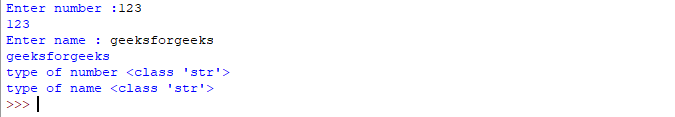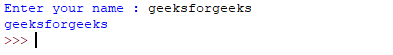# Taking input in Python

• Difficulty Level : Easy
• Last Updated : 12 May, 2022

Developers often have a need to interact with users, either to get data or to provide some sort of result. Most programs today use a dialog box as a way of asking the user to provide some type of input. While Python provides us with two inbuilt functions to read the input from the keyboard.• input ( prompt )
• raw_input ( prompt )

input ( ) : This function first takes the input from the user and convert it into string. Type of the returned object always will be <type ‘str’>. It does not evaluate the expression it just return the complete statement as String. For example –

## Python3

 `# Python program showing  ` `# a use of input() ` ` `  `val ``=` `input``(``"Enter your value: "``) ` `print``(val) `

Output:How the input function works in Python :

• When input() function executes program flow will be stopped until the user has given an input.
• The text or message display on the output screen to ask a user to enter input value is optional i.e. the prompt, will be printed on the screen is optional.
• Whatever you enter as input, input function convert it into a string. if you enter an integer value still input() function convert it into a string. You need to explicitly convert it into an integer in your code using typecasting

Code:

## Python3

 `# Program to check input  ` `# type in Python ` ` `  `num ``=` `input` `(``"Enter number :"``) ` `print``(num) ` `name1 ``=` `input``(``"Enter name : "``) ` `print``(name1) ` ` `  `# Printing type of input value ` `print` `(``"type of number"``, ``type``(num)) ` `print` `(``"type of name"``, ``type``(name1)) `

Output :raw_input ( ) : This function works in older version (like Python 2.x). This function takes exactly what is typed from the keyboard, convert it to string and then return it to the variable in which we want to store. For example –

## Python

 `# Python program showing  ` `# a use of raw_input() ` ` `  `g ``=` `raw_input``(``"Enter your name : "``) ` `print` `g `

Output :Here, g is a variable which will get the string value, typed by user during the execution of program. Typing of data for the raw_input() function is terminated by enter key. We can use raw_input() to enter numeric data also. In that case we use typecasting.For more details on typecasting refer this

Refer to the article Taking list as input from the user for more information.

My Personal Notes arrow_drop_up
Recommended Articles
Page :